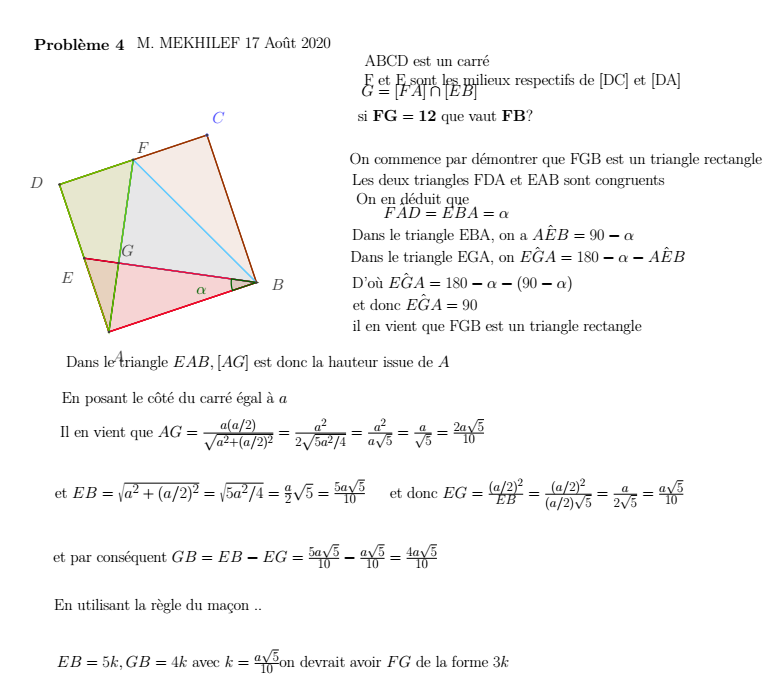# pgfplot error while compiling

mounib.mekhilef shared this question 2 years ago

Hi there

I never managed to compile the exported LaTeX figures !

Here is what I get (the picture actually is just a circle) see screenshot.

Anyone have been thru? any option/explanation?1\documentclass[10pt]{standalone}
\usepackage{pgf,tikz}
\pagestyle{empty}
\begin{document}
\newrgbcolor{ududff}{0.30196078431372547 0.30196078431372547 1}
\newrgbcolor{zzttqq}{0.6 0.2 0}
\newrgbcolor{wwccff}{0.4 0.8 1}
\newrgbcolor{ffqqtt}{1 0 0.2}
\newrgbcolor{wwccqq}{0.4 0.8 0}
\newrgbcolor{qqwuqq}{0 0.39215686274509803 0}
\psset{xunit=1cm,yunit=1cm,algebraic=true,dimen=middle,dotstyle=o,dotsize=5pt 0,linewidth=2pt,arrowsize=3pt 2,arrowinset=0.25}
\begin{pspicture*}(-23.78649633016404,-26.63706409275791)(30.911558744839667,12.806005772885314)
\Huge
\pspolygon[linewidth=2pt,linecolor=zzttqq,fillcolor=zzttqq,fillstyle=solid,opacity=0.1](-14.941332650675266,0.7381721749647143)(-6.911827825035284,3.431332650675268)(-4.218667349324733,-4.598172174964715)(-12.248172174964715,-7.291332650675267)
\pspolygon[linewidth=2pt,linecolor=wwccff,fillcolor=wwccff,fillstyle=solid,opacity=0.1](-10.926580237855275,2.0847524128199915)(-11.71953540012094,-3.5408986252771637)(-4.218667349324733,-4.598172174964715)
\pspolygon[linewidth=2pt,linecolor=ffqqtt,fillcolor=ffqqtt,fillstyle=solid,opacity=0.1](-13.59475241281999,-3.2765802378552764)(-12.248172174964715,-7.291332650675267)(-4.218667349324733,-4.598172174964715)
\pspolygon[linewidth=2pt,linecolor=wwccqq,fillcolor=wwccqq,fillstyle=solid,opacity=0.1](-10.926580237855275,2.0847524128199915)(-14.941332650675266,0.7381721749647143)(-12.248172174964715,-7.291332650675267)
\psline[linewidth=2pt,linecolor=zzttqq](-14.941332650675266,0.7381721749647143)(-6.911827825035284,3.431332650675268)
\psline[linewidth=2pt,linecolor=zzttqq](-6.911827825035284,3.431332650675268)(-4.218667349324733,-4.598172174964715)
\psline[linewidth=2pt,linecolor=zzttqq](-4.218667349324733,-4.598172174964715)(-12.248172174964715,-7.291332650675267)
\psline[linewidth=2pt,linecolor=zzttqq](-12.248172174964715,-7.291332650675267)(-14.941332650675266,0.7381721749647143)
\psline[linewidth=2pt](-10.926580237855275,2.0847524128199915)(-12.248172174964715,-7.291332650675267)
\psline[linewidth=2pt](-13.59475241281999,-3.2765802378552764)(-4.218667349324733,-4.598172174964715)
\rput[tl](1.6842897851643188,7.8126322358875075){ABCD est un carré}
\rput[tl](1.6384790187698433,6.804795375209051){F et E sont les milieux respectifs de [DC] et [DA]}
\rput[tl](1.501046719586417,6.209255412080872){$G = [FA] \cap [EB]$}
\rput[tl](1.317803654008515,4.789121653852138){$\mathbf{\mbox{si } FG=12 \mbox{ que vaut } FB ?}$}
\rput[tl](-16.319341407864542,8.683036797382538){\textbf{Problème 4}}
\psline[linewidth=2pt,linecolor=wwccff](-10.926580237855275,2.0847524128199915)(-11.71953540012094,-3.5408986252771637)
\psline[linewidth=2pt,linecolor=wwccff](-11.71953540012094,-3.5408986252771637)(-4.218667349324733,-4.598172174964715)
\psline[linewidth=2pt,linecolor=wwccff](-4.218667349324733,-4.598172174964715)(-10.926580237855275,2.0847524128199915)
\psline[linewidth=2pt,linecolor=ffqqtt](-13.59475241281999,-3.2765802378552764)(-12.248172174964715,-7.291332650675267)
\psline[linewidth=2pt,linecolor=ffqqtt](-12.248172174964715,-7.291332650675267)(-4.218667349324733,-4.598172174964715)
\psline[linewidth=2pt,linecolor=ffqqtt](-4.218667349324733,-4.598172174964715)(-13.59475241281999,-3.2765802378552764)
\psline[linewidth=2pt,linecolor=wwccqq](-10.926580237855275,2.0847524128199915)(-14.941332650675266,0.7381721749647143)
\psline[linewidth=2pt,linecolor=wwccqq](-14.941332650675266,0.7381721749647143)(-12.248172174964715,-7.291332650675267)
\psline[linewidth=2pt,linecolor=wwccqq](-12.248172174964715,-7.291332650675267)(-10.926580237855275,2.0847524128199915)
\rput[tl](0.8596959900637603,2.452772567733899){On commence par démontrer que FGB est un triangle rectangle}
\rput[tl](0.9971282892471868,1.3075034078720167){Les deux triangles FDA et EAB sont congruents}
\rput[tl](1.226182121219564,0.2996665471935603){On en déduit que}
\rput[tl](2.6921266458427793,-0.2500626495401432){$\hat{FAD} = \hat{EBA} =\alpha$}
\rput[tl](0.9971282892471868,-1.4411425757965006){$\mbox{Dans le triangle EBA, on a } \hat{AEB}=90°-\alpha$}
\pscustom[linewidth=2pt,linecolor=qqwuqq,fillcolor=qqwuqq,fillstyle=solid,opacity=0.1]{
\parametricplot{3.0015616713492927}{3.465209280350099}{1.374322991834264*cos(t)+-4.218667349324733|1.374322991834264*sin(t)+-4.598172174964715}
\lineto(-4.218667349324733,-4.598172174964715)\closepath}
\rput[tl](0.9055067564582358,-2.6780332684473334){$\mbox{Dans le triangle EGA, on } \hat{EGA}=180°-\alpha - \hat{AEB}$}
\rput[tl](0.9971282892471868,-4.052356260281592){$\mbox{D'où } \hat{EGA}=180°-\alpha - (90°-\alpha)$}
\rput[tl](1.0429390556416622,-5.2434361865379495){$\mbox{et donc } \hat{EGA}=90°$}
\rput[tl](0.7680744572748094,-6.617759178372208){$\mbox{ il en vient que FGB est un triangle rectangle}$}
\rput[tl](-14.578532284874473,-8.54181136694017){$\mbox{Dans le triangle } EAB, [AG] \mbox{ est donc la hauteur issue de } A$}
\rput[tl](-14.899207649635802,-12.023429612920292){$\mbox{Il en vient que } AG=\frac{a (a/2)}{\sqrt{a^2+(a/2)^2}}=\frac{a^2}{2\sqrt{5a^2/4}}=\frac{a^2}{a\sqrt{5}}=\frac{a}{\sqrt{5}}=\frac{2a\sqrt{5}}{10}$}
\rput[tl](-14.80758611684685,-10.557485088297083){$\mbox{En posant le côté du carré égal à } a$}
\rput[tl](-15.174072248002654,-15.321804793322514){$\mbox{et } EB=\sqrt{a^2+(a/2)^2}=\sqrt{5a^2/4}=\frac{a}{2}\sqrt{5}=\frac{5a\sqrt{5}}{10}$}
\rput[tl](3.058612776998583,-15.275994026928037){$\mbox{et donc } EG=\frac{(a/2)^2}{EB}=\frac{(a/2)^2}{(a/2) \sqrt{5}}=\frac{a}{2\sqrt{5}}=\frac{a\sqrt{5}}{10}$}
\rput[tl](-15.265693780791604,-18.84923380569711){$\mbox{et par conséquent } GB=EB-EG=\frac{5a\sqrt{5}}{10}-\frac{a\sqrt{5}}{10}=\frac{4a\sqrt{5}}{10}$}
\rput[tl](-15.21988301439713,-21.826933621338004){$\mbox{En utilisant la règle du maçon ..}$}
\rput[tl](-15.082450715213703,-24.575579605006524){$EB=5k, GB=4k \mbox{ avec } k=\frac{a\sqrt{5}}{10} \mbox{on devrait avoir } FG \mbox{ de la forme } 3k$}
\rput[tl](-15.403126079975031,-28.057197850986647){$\mbox{et } FG=FA-AG=EB-AG=\frac{5a\sqrt{5}}{10}-\frac{2a\sqrt{5}}{10}=\frac{3a\sqrt{5}}{10}$}
\rput[tl](-15.21988301439713,-32.592463724039696){$\mbox{puisque } FG=12 \mbox{ il en vient que }$}
\rput[tl](1.821722084347745,-31.355573031388868){$\frac{3a\sqrt{5}}{10}=12 \mbox{ et donc } a=\frac{40}{\sqrt{5}}$}
\rput[tl](-15.31150454718608,-36.53218963396458){$\mbox{On en déduit donc que } FB=EB=\frac{5\sqrt{5}}{10} \frac{40}{\sqrt{5}}=20$}
\rput[tl](-14.80758611684685,-40.5635370766784){\textbf{Vérification}}
\rput[tl](-13.662316956984963,-42.166913900485035){$FG^2 + GB^2=FB^2$}
\rput[tl](-13.433263125012585,-45.55691061367621){$\rightarrow (\frac{3a\sqrt{5}}{10})^ 2+ (\frac{4a\sqrt{5}}{10})^2= (\frac{5a\sqrt{5}}{10})^2$}
\rput[tl](-13.29583082582916,-49.175961158839755){$\rightarrow 3^2 + 4^2 = 5^2$}
\rput[tl](-12.746101629095454,-52.7950117040033){$9+16=25$}
\rput[tl](-5.966108202713085,-52.6117686384254){$\mbox{Ce qui est donc vérifié}$}
\rput[tl](-10.730427907738534,8.774658330171489){M. MEKHILEF 17 Août 2020}

\psdots[dotstyle=*,linecolor=ududff](-6.911827825035284,3.431332650675268)
\rput[bl](-6.607458932235741,4.101960157935009){\ududff{$C$}}
\psdots[dotsize=4pt 0,dotstyle=*,linecolor=darkgray](-4.218667349324733,-4.598172174964715)
\rput[bl](-3.4007052846224584,-5.014382354565573){\darkgray{$B$}}
\psdots[dotsize=4pt 0,dotstyle=*,linecolor=darkgray](-14.941332650675266,0.7381721749647143)
\rput[bl](-16.548395239836918,0.5287203791659367){\darkgray{$D$}}
\psdots[dotsize=4pt 0,dotstyle=*,linecolor=darkgray](-6.911827825035284,3.431332650675268)
\psdots[dotsize=4pt 0,dotstyle=*,linecolor=darkgray](-12.248172174964715,-7.291332650675267)
\rput[bl](-12.058940133178321,-8.908297498095973){\darkgray{$A$}}
\psdots[dotsize=4pt 0,dotstyle=*,linecolor=darkgray](-10.926580237855275,2.0847524128199915)
\rput[bl](-10.730427907738534,2.452772567733899){\darkgray{$F$}}
\psdots[dotsize=4pt 0,dotstyle=*,linecolor=darkgray](-13.59475241281999,-3.2765802378552764)
\rput[bl](-14.853396883241325,-4.602085457015296){\darkgray{$E$}}
\psdots[dotsize=4pt 0,dotstyle=*,linecolor=darkgray](-11.71953540012094,-3.5408986252771637)
\rput[bl](-11.555021702839092,-3.1819516987865617){\darkgray{$G$}}
\rput[bl](-7.477863493730775,-5.2434361865379495){\qqwuqq{$\alpha$}}

\end{pspicture*}
\end{document}1

Also there are some really good hints from -Loco- here

https://help.geogebra.org/t...